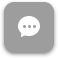Call Now 855-300-1469

# EBA 3334 Applied Decision Methods for Business

#### Course Description

Uses quantitative techniques to aid in decision making. Emphasizes problem identification and applies appropriate solution techniques for interpretation of results. Includes probability theory, decision making under certainty, risk and uncertainty, inventory control, forecasting, PERT/CPM, utility theory, and linear programming.

#### Course Objectives

After completing this course, students will be able to

• Discuss how management science models are created and used to improve managerial decisions in business
• Explain the key quantitative methods used in diverse management science models
• Identify the strengths and limitations of the key quantitative methods
• Select and apply the method most appropriate to the problem at hand

### Week 1

##### Lecture: Linear Programming Part One

Outcomes

• Define management science
• Describe the management science approach to problem solving
• Discuss the nature of a model
• Differentiate between normative model results and descriptive model results
• Identify the basic components of break-even analysis
• Demonstrate break-even analysis
• Describe linear programming
• Design a linear programming model
• Explain what a maximization linear programming model is
• Find a graphical solution to a maximization linear programming model
• Apply such a model in the appropriate managerial decision situations

### Week 2

##### Lecture: Transportation Problem Part One

Outcomes

• Explain what a maximization linear programming model is
• Produce a graphical solution to a maximization linear programming model
• Apply such a model in the appropriate managerial decision situations
• Describe the different types of irregular linear programming models
• Analyze situations when encountering an irregular linear programming model
• Convert constraints in linear programming models
• Define the standard form of a linear programming model
• Convert problems into the proper form for computer solution
• Solve linear programming problems using spreadsheets
• Define transportation problems
• Describe the connection between transportation problems and linear programming problems
• Solve transportation problems using the Northwest Corner method
• Solve transportation problems using the minimum cell cost method
• Solve transportation problems using Vogel's Approximation Method

### Week 3

##### Lecture: Project Management

Outcomes

• Solve transportation problems using the stepping-stone method
• Describe an unbalanced transportation problem
• Solve unbalanced transportation problems
• Describe degeneracy in transportation problems
• Solve degenerate transportation problems
• Define network flow problems
• Use the shortest route solution approach
• Use the minimal spanning tree solution approach
• Solve the maximal flow problem
• Describe the elements of project management
• Describe the project network
• Discuss the concept of critical path
• Solve project management problems using CPM

### Week 4

##### Lecture: Markov Analysis

Outcomes

• Describe the fundamentals of probability theory
• Discuss the issues of statistical independence and dependence
• Use expected values
• Apply the normal distribution
• Describe the type of systems that are suited to a Markov analysis
• Determine the characteristics of a Markov analysis
• Solve problems using Markov analysis

### Week 5

##### Lecture: Queuing Analysis

Outcomes

• Describe the type of systems that are suited to a queuing analysis
• Describe the characteristics of a queuing analysis
• Solve problems using queuing analysis
• Describe the concept of forecasting
• Discuss the components of forecasting
• Use the moving average forecasting method
• Use the weighted average forecasting method
• Use the exponential smoothing method
• Use the adjusted exponential smoothing method
• Describe the EOQ model using noninstantaneous receipt
• Discuss the concept of reorder point
• Determine safety stocks using service levels

### Week 6

##### Lecture: Inventory Management

Outcomes

• Describe the elements of inventory management
• Discuss the different types of inventory control systems
• Use the Economic Order Quantity (EOQ) model
• Describe the EOQ model with noninstantaneous receipt
• Discuss the concept of reorder point
• Determine safety stocks using service levels

### Week 7

##### Lecture: Decision Analysis

Outcomes

• Discuss the key categories of decision situations
• Define the components of decision making
• Use the maximax criterion method
• Use the maximin criterion method
• Use the minimax regret criterion method
• Use the Hurwicz criterion method
• Use the equal likelihood criterion method
• Discuss decision making with probabilities
• Apply the concept of expected value
• Use the expected value to make decisions
• Use decision trees to make decisions

### Week 8

##### Lecture: Game Theory

Outcomes

• Define game theory
• Discuss the major types of games
• Apply techniques in pure strategy games to make decisions
• Apply techniques in mixed strategy games to make decisions

The course description, objectives and learning outcomes are subject to change without notice based on enhancements made to the course. December 2013